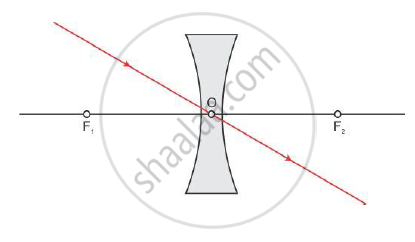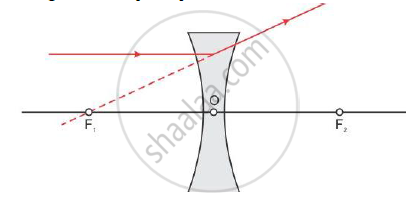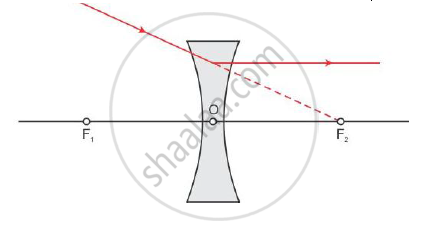# Draw a Ray Diagram to Show the Path of the Refracted Ray in Each of the Following Cases:- a Ray of Light Incident on a Concave Lens is - Science

Draw a ray diagram to show the path of the refracted ray in each of the following cases:-

A ray of light incident on a concave lens is

(i) passing through its optical centre.

(ii) parallel to its principal axis.

(iii) directed towards its principal focus.

#### Solution

(i) A ray of light passing through the optical centre of the concave lens will emerge without any deviation.(ii) A ray of light parallel to the principal axis, after refraction from a concave lens, appears to diverge from the principal focus on the same side of the lens.(iii) A ray of light directed towards the principal focus of a concave lens, becomes parallel to its principal axis after refraction through the lens.Concept: Concave Mirror
Is there an error in this question or solution?